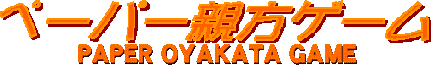### Daily results

### Day 15 results

Average = 24.8 pt.  Highest = 47 pt.  Lowest = 4 pt.
Daily top = lemon  Rank up = Pandaazuma ( 32 persons )
Perfect =
Semi perfect =
Reverse Perfect =

### Day 14 results

Average = 22.4 pt.  Highest = 42 pt.  Lowest = 1 pt.
Daily top = dai  Rank up = dai ( 29 persons )
Perfect =
Semi perfect =
Reverse Perfect =

### Day 13 results

Average = 29.4 pt.  Highest = 47 pt.  Lowest = 8 pt.
Daily top = mura  Rank up = Kaito ( 29 persons )
Perfect =
Semi perfect =
Reverse Perfect =

### Day 12 results

Average = 39.1 pt.  Highest = 67 pt.  Lowest = 10 pt.
Daily top = bill, fusen  Rank up = bill ( 33 persons )
Perfect =
Semi perfect = bill, fusen
Reverse Perfect =

### Day 11 results

Average = 34.9 pt.  Highest = 61 pt.  Lowest = 13 pt.
Daily top = KANSAIOITEKAZE  Rank up = wakaiouzenjou ( 52 persons )
Perfect =
Semi perfect = KANSAIOITEKAZE, wakaiouzenjou
Reverse Perfect =

### Day 10 results

Average = 24.4 pt.  Highest = 44 pt.  Lowest = 3 pt.
Daily top = Beeftank  Rank up = Beeftank ( 46 persons )
Perfect =
Semi perfect =
Reverse Perfect =

### Day 9 results

Average = 31.9 pt.  Highest = 49 pt.  Lowest = 16 pt.
Daily top = SENTOREA, tsukihikari  Rank up = kouteiou ( 34 persons )
Perfect =
Semi perfect =
Reverse Perfect =

### Day 8 results

Average = 44.6 pt.  Highest = 74 pt.  Lowest = 19 pt.
Daily top = miyabi  Rank up = fuurinkazan ( 75 persons )
Perfect =
Semi perfect = kitakachiyama, Ufoshin, lake, Asapedroryu, Andoreasu, WASAKIGAWA, choshu-yuki, suitenguuyama, yamanoyama, miyabi, fuurinkazan, Golynohana, marihime
Reverse Perfect =

### Day 7 results

Average = 40.3 pt.  Highest = 72 pt.  Lowest = 14 pt.
Daily top = fujisan  Rank up = fujisan ( 93 persons )
Perfect =
Semi perfect = fujisan
Reverse Perfect =

### Day 6 results

Average = 36.2 pt.  Highest = 61 pt.  Lowest = 9 pt.
Daily top = Kanpei  Rank up = gijou ( 63 persons )
Perfect =
Semi perfect =
Reverse Perfect =

### Day 5 results

Average = 24.1 pt.  Highest = 46 pt.  Lowest = 6 pt.
Daily top = marionette  Rank up = Kaito ( 58 persons )
Perfect =
Semi perfect =
Reverse Perfect =

### Day 4 results

Average = 35.7 pt.  Highest = 60 pt.  Lowest = 9 pt.
Daily top = raitest, sana  Rank up = Terarno ( 70 persons )
Perfect =
Semi perfect = Terarno
Reverse Perfect =

### Day 3 results

Average = 27.8 pt.  Highest = 60 pt.  Lowest = 8 pt.
Daily top = express  Rank up = Konosato ( 76 persons )
Perfect =
Semi perfect = express
Reverse Perfect =

### Day 2 results

Average = 24.5 pt.  Highest = 45 pt.  Lowest = 1 pt.
Daily top = nekoyanagi  Rank up = GONZABUROW ( 83 persons )
Perfect =
Semi perfect =
Reverse Perfect =

### Day 1 results

Average = 28.3 pt.  Highest = 48 pt.  Lowest = 6 pt.
Daily top = joaoiyama
Perfect =
Semi perfect =
Reverse Perfect =